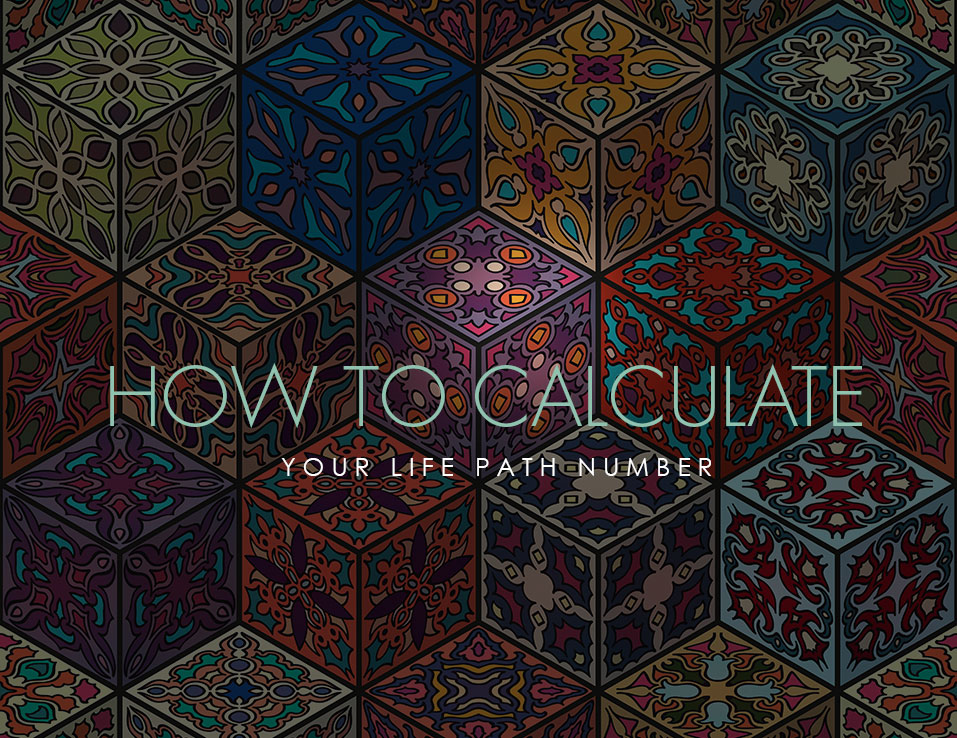## 20 Aug How to calculate your life path number

The question I get asked all the time is “how do I calculate my life path number”?

First, the life path number gives you information about your destiny and what you came here to do, learn, and accomplish. It is considered the most important number in your numerology cosmos.

I always calculate to see if you have a double digit (not all birthdays add up to have a double digit) because it provides more detail on what your life path number is. For example, life path numbers 17/8  and 26/8 have subtle important difference even though the underlining properties are the same.  A 26/8  will have more focus on family,  children, loved ones, and the home life as it pertains to money, finances, power, and structure. Knowing this is important, especially if you have been dealing with issues on compromising the needs of the family financially vs your own needs.

### EXAMPLE 1

Say your birthday is – 3/17/1980
First add the year – 1 + 9 + 8 + 0 = 18 and 1+8 = 9
Then calculate the day you were born – 1 + 7 = 8
Next calculate the month = 3

Then add them all together 3 + 8 + 9 = 20/2 (note the double digit has meaning so this person has a life path number of 20 or 2)

### EXAMPLE 2

If you have master numbers in your birthdays such as 11 or 22 you do not break those down when adding them because they have specific meanings and they will give you a different life path number as it pertains to the double digit. For example.

Say our birthday is – 11/8/1979
First add the year – 1+9+7+9 = 26 = 2+6 = 8
Since the day is a single digit it just = 8

And since 11 is a master number you do not break this down to 1 + 1 = 2 you actually keep it as 11

So now add 11+8+8 = 27/9

### EXAMPLE 3

Say your birthday is – 6/22/1984
First add the year – 1+9+8+4 = 22 (do not break this down to 4 because it is a master number)
Since the day is also a master number you do not break this down = 22
Month = 6

So now add – 6+22+22 = 50/5

Hope this has not confused you too much! And cheers if it has helped you understand how to calculate your life path number!nicholescosmiccove@gmail.com